# Glossary

Definitions of common terms in data science and ML monitoring.

### Accuracy

Accuracy is the measure of the number of correct predictions made by the model. It is derived by calculating the percentage of correct predictions out of overall predictions. accuracy = correct predictions / all predictions
Accuracy = Correct Predictions / All Predictions
Example: There are 100 credit card transactions; 90 transactions are legitimate and 10 transactions are fraudulent. If your model predicts that 95 transactions are legitimate and 5 transactions are fraudulent, its accuracy is:
95% = (90 correct legitimate + 5 correct fraudulent) / 100 transactions

### Baseline

A baseline is the reference data or benchmark used to compare model performance against for monitoring purposes. Baselines can be training data, validation data, prior time periods of production data, a previous model version, among others.

### Baseline Distribution

A baseline distribution refers to a model dataset used as a reference or comparison to the modelвАЩs current (production) distribution. Baseline distributions in Arize AIвАЩs platform can be training datasets, validation datasets, or prior time periods of production.

### Binary Classification Model

Binary classification refers to machine learning algorithms that have classification tasks that have only and only two class labels. Binary classification involves one вАЬpositiveвАЭ and one вАЬnegativeвАЭ class state in general.

### Binning

Data Binning is a way to group a number of continuous values together into smaller cohorts or вАЬbinsвАЭ. The technique helps reduce the cardinality of data by representing the points in intervals -- for example, age ranges.

### Calibration

вАЛCalibration is the comparison of the actual output and the expected output given by a system.
Example: Let's say we are predicting credit card fraud and produce the following prediction/actual pairs (0.1, 0.1), (0.2, 0.1), (0.9, 0.7).
Our calibration calculation is as follows:
calibration = average prediction / average actual
= ((0.1 + 0.2 + 0.9)/3) / ((0.1 + 0.1 + 0.7)/3) = 0.4 / 0.3
 = 1.333
In this case we see we are on average predicting higher (more fraud) than the ground truth.

### Canary Deployment

A method of testing a new model or model version where only a small subset of production data flows through this model to verify response performance before making a complete cutover. This technique allows for deeper analysis and understanding of model behavior and can minimize risk that regressions severely impact the business or customers.

### Cardinality - new and missing values

Cardinality of new and missing values is a measurement of the number of unique values in one dataset that do not appear in another. For new values, it counts the number of unique values in production that don't appear in the baseline. For missing values, it counts the unique values in the baseline that don't appear in production.
Assume you have an insurance claims model with a feature for claim type. Their unique values are below:
production_claims = {'auto', 'home', 'car'}
baseline_claims = {'auto', 'home', 'personal'}
The calculation for new values would be as such:
new_values = production_claims - baseline_claims = {'car'}
count_new_values = len(new_values) = 1
You can see that production has a new value compared to the baseline - car. If your baseline is training, this could indicate that your model is seeing an unexpected value in production and may have issues.
The calculation for missing values is the reverse of new values:
missing_values = baseline_claims - production_claims = {'personal'}
count_missing_values = len(missing_values) = 1
In this case, production is missing the value personal. This could be normal - it's possible that the company no longer supports this type of claim but the model was trained on a past period when it did.
This type of metric is different than purely using cardinality. In this example, both datasets have a cardinality of 3, making it seem like there is no issue.
Note that the monitor will alert on the count of new values or the count of missing values. The monitor will also show a list of the actual values that are new or missing.

### Classification Model

Classification models are used to predict categories or assign a class label. Any given data is classified into a set of categories or groups to determine its further use or for processing needs.
Data that can be classified into one category or a second category is known as binary data. For example, fraud or not fraud, male or female.
If the set of data can be classified into a number of categories or groups, each based on a different criterion, such data is known as multi-class data. For example, education level, household income.

### Confusion Matrix

A confusion matrix provides a summary of all prediction results of a classification problem. Each result is shown with its corresponding number of correct/incorrect predictions (see True Positive, True Negative, False Positive, False Negative), count values and classification criteria. By providing a neat summary of all possible results, the confusion matrix lets you know the ways your classification model could get confused when making the predictions. It helps identify errors and the type of errors made by the model and thus helps improve the accuracy of the classification model.

### Current Distribution

Current Distribution refers to the statistical distribution, or shape, of the dataset being generated by a machine learning model in production. Distribution of datasets in machine learning models are represented in the form of functions that show the relationships between the various observations, visually presented in the form of curves or graphs.

### Data Quality

Data quality refers to the integrity and consistency of the data sets used. In monitoring machine learning performance, data quality measures include attributes such as missingness, out of range, P1 and P99, type mismatch, among others.

### Deep Learning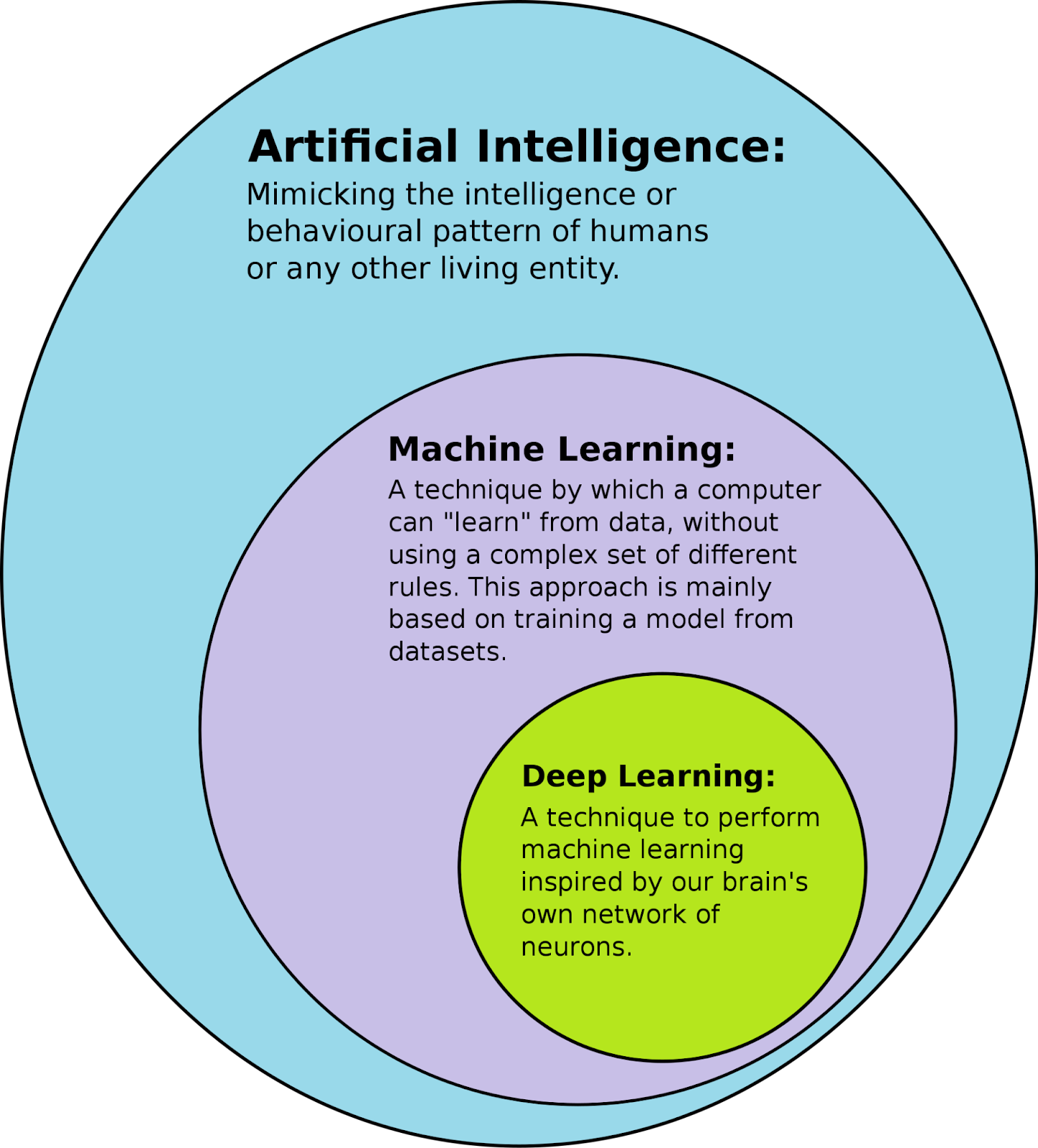Machine learning is a subset of AI, and it consists of the techniques that enable computers to figure things out from the data and deliver AI applications. Deep learning, meanwhile, is a subset of machine learning that enables computers to solve more complex problems inspired by the human brainвАЩs network of neurons.

### Deep Learning Model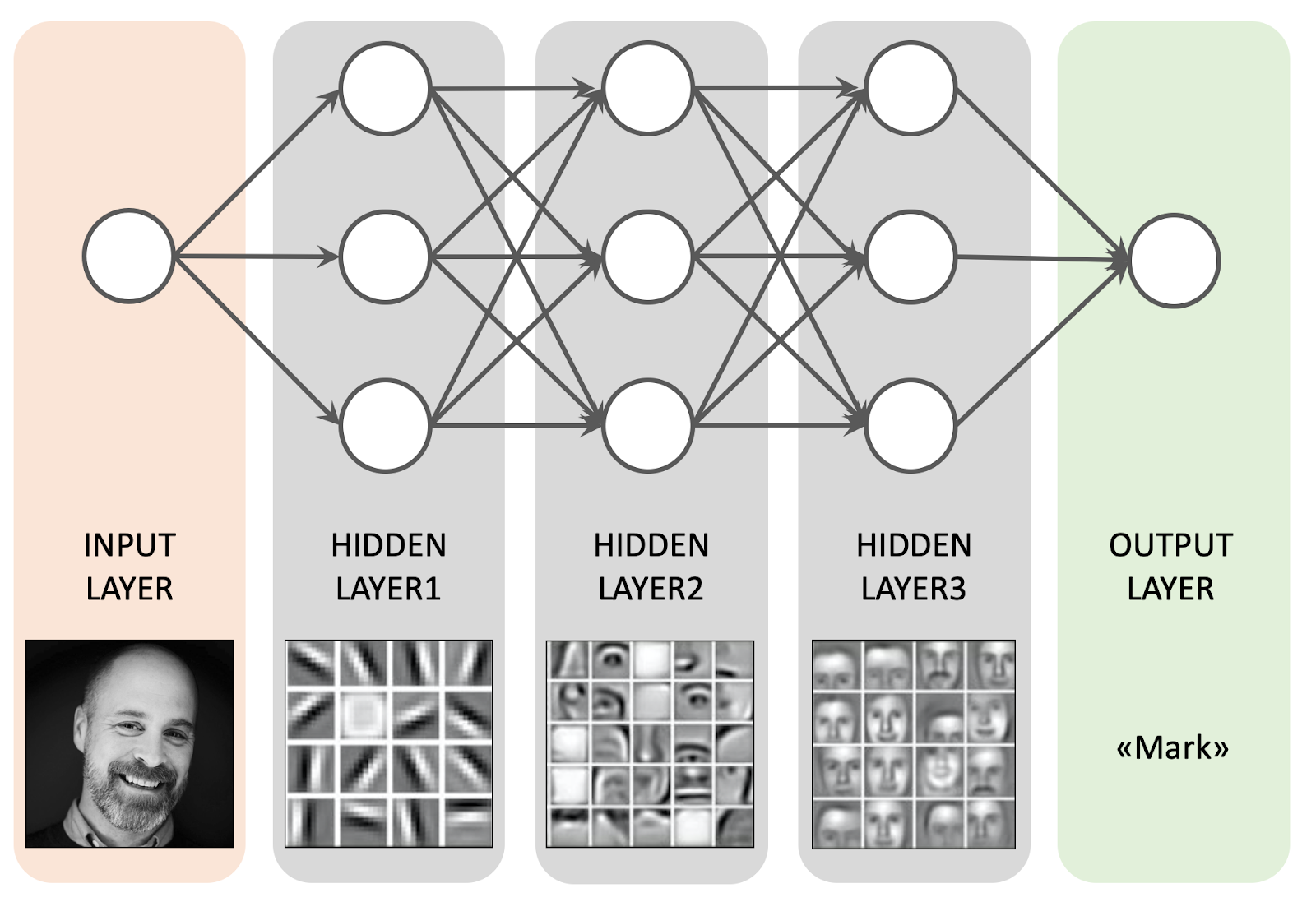A deep learning model normally refers to a neural network, typically with more than two layers). Deep learning is usually used for computationally dense tasks like computer vision (images) and natural language processing.

### Disparate Impact

Disparate Impact (also called adverse impact) is a quantitative measure of the adverse treatment of protected classes. The calculation is the proportion of the sensitive group that received the positive outcome divided by the proportion of the base group that received the positive outcome.
Disparate impact in United States labor law refers to practices in employment, housing, and other areas that adversely affect one group of people of a protected characteristic more than another, even though rules applied by employers or landlords are formally neutral. Although the protected classes vary by statute, most federal civil rights laws protect based on race, color, religion, national origin, and sex as protected traits, and some laws include disability status and other traits as well. (source: Wikipedia)### Drift

Drift is defined as the change in the data over time. It also means the change in the properties of the target variable, due to unpredictable or unforeseen changes, over the due course of time.
• Data drift can be described as the change in the distribution of data, between the real-time data and the baseline data that was predicted or set beforehand.
• Concept drift is the change between the relationship between input and the output given in any situation.
Drift can be in any form. It can be gradual, recurring, or sudden. It can be a positive or negative drift. The change in data over time can affect model outcomes, making drift an important metric to monitor when it comes to model performance.

### Embedding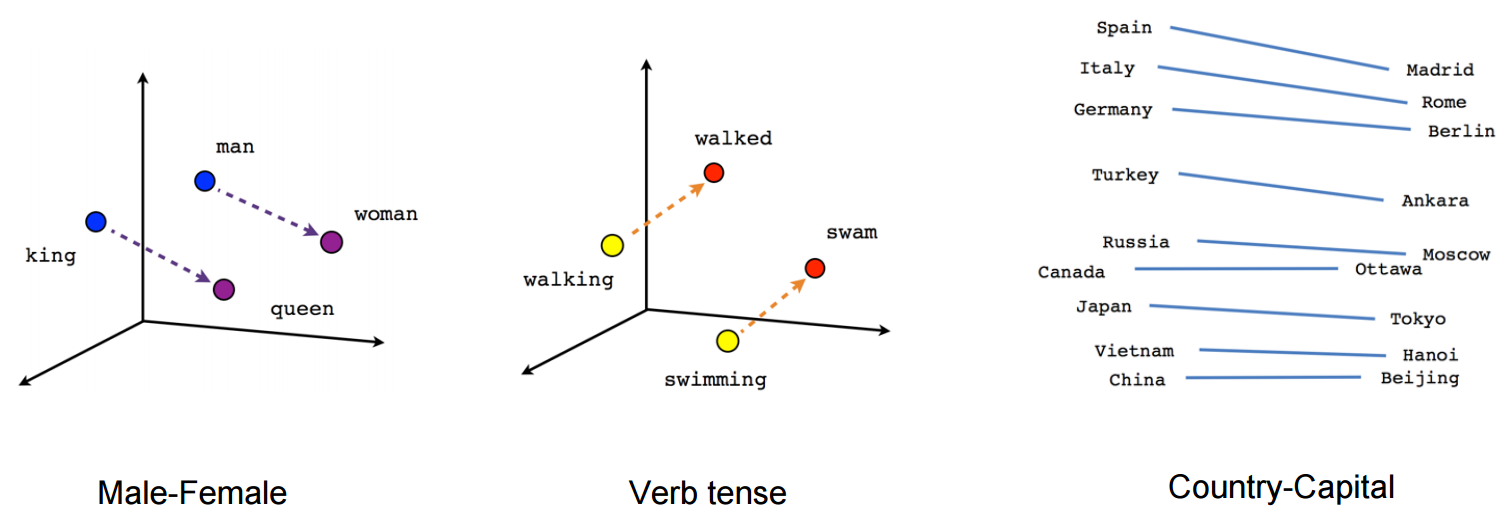In natural language processing (see definition of вАШnatural language processing), embedding is a term used for the representation of words for text analysis, typically in the form of a real-valued vector that encodes the meaning of the word such that the words that are closer in the vector space are expected to be similar in meaning.

### Euclidean Distance

вАЛEuclidean distance is calculated as the square root of the sum of the squared differences between the components of two vectors.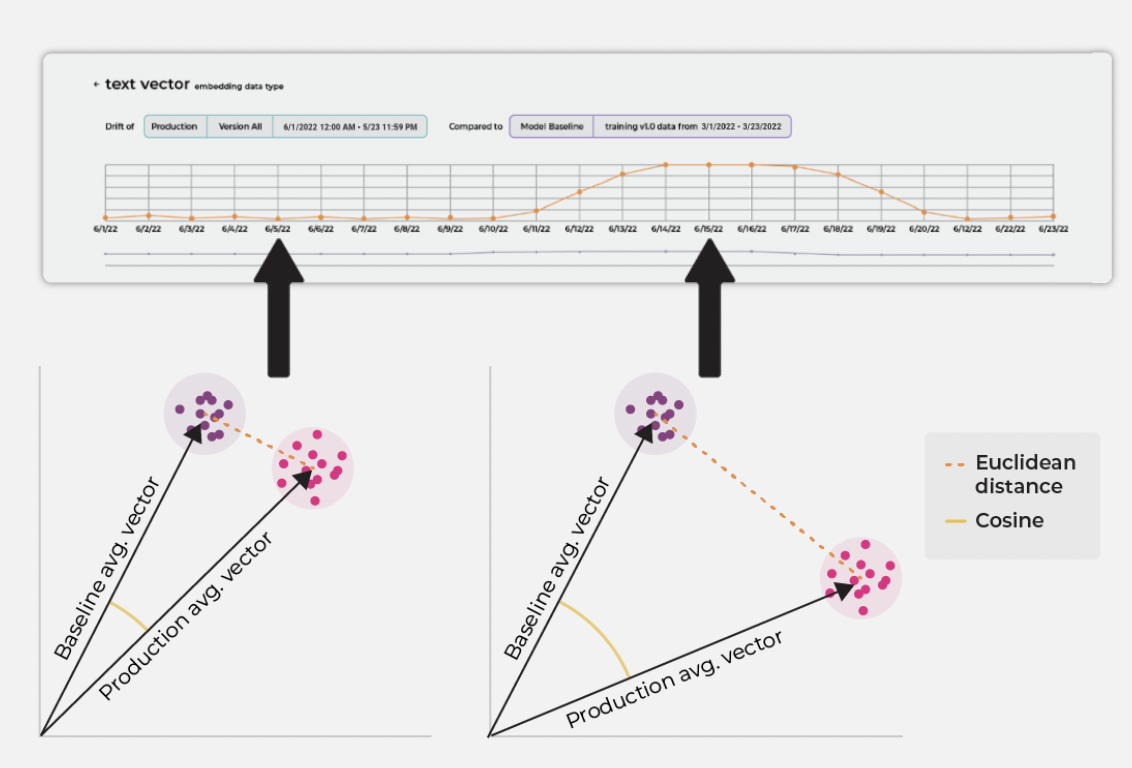We can use Euclidean distance (like in the image above), to compare two sets of embeddings. We can calculate the centroids of the two sets to see if one centroid may be drifting relative to the other using the Euclidean distance.
An example of Euclidean distance between two centroids, x and y, is given below for 2D, 3D, and N-dimensional vectors:
$Distance_{2D} = \sqrt{(x_1 - y_1)^2 + (x_2 - y_2)^2}$
$Distance_{3D} = \sqrt{(x_1 - y_1)^2 + (x_2 - y_2)^2 + (x_3 - y_3)^2}$
$Distance_{N_D} = \sqrt{\sum_{i=1}^{N}(x_i - y_i)^2}$

### Evaluation Metric

The way the performance of a predictive model is quantified and calculated is known as the evaluation metric. It is used to evaluate the accuracy and the performance of the model used.

### Evaluation Store / Inference Store

A machine learning infrastructure tool to monitor and improve model performance. Think of them as the ledger or log of model activities/inferences. Evaluation stores are used to:
• Surface up performance metrics in aggregate (or slice) for any model, in any environmentвАКвАФвАКproduction, validation, training
• Monitor and identify drift, data quality issues, or anomalous performance degradations using baselines
• Enable teams to connect changes in performance to why they occurred
• Provide a platform to help deliver models continuously with high quality and feedback loops for improvementвАКвАФвАКcompare production to training
• Provide an experimentation platform to A/B test model versions

### Evaluation Window

The evaluation window is plot of the period or duration of time against the metric being calculated. For instance, the previous 30 days. Any evaluation metric that can be represented as a duration of time can be visualised as an evaluation window.

### Explainability

вАЛExplainability is defined as the total extent to which the machine learning internal mechanics can be explained in human-understandable terms only. It is simply the process of explaining the reasons behind the machine learning aspects of output data (see definition, вАШSHAPвАЩ).

### F-score

Measure of the harmonic mean of precision and recall. F-score is a result of integrating these parameters into one for a better understanding of the accuracy of the model. F-score can be modified into F, 0.5, 1, & 2 based on the measure of weightage given to precision over recall.

### False Negative

When a model mistakenly predicts a negative class, when the value belongs to the positive class.
Example: A model flags a credit card transaction as вАШnot fraudвАЩ when it is actually a fraudulent transaction.

### False Positive

When a model mistakenly predicts a positive class, when the value belongs to the negative class.
Example: A model flags a credit card transaction as вАШfraudвАЩ when it was not actually a fraudulent transaction.

### False Positive Rate

False Positive Rate (FPR) is a the rate of "false alarms". In other words, it is a measure of the probability a true negative will be missed.

### False Positive Rate Parity

False Positive Rate Parity is a measure of the False Positive Rate (FPR) of a sensitive group as compared to a base group.### Feature

Feature is a term of art for inputs into a model. The label can be used to mean a couple things and are a bit more fluid in use. Normally a label is a target of a prediction but can sometimes be the output of a model. A target of a prediction also can be known as ground truth.

### Feature Importance

Feature importance is a compilation of a class of techniques that take in all the features related to making a model prediction and assign a certain score to each feature to weigh how much or how little it impacted the outcome. These scores can then be used to better understand the internal logic of a model, make necessary changes to the model to improve its accuracy, and also reduce unnecessary inputs.

### Feature Performance Heat Map

A feature performance heat map is a visual representation of the performance of each feature in a given model. It enables users to quickly see slices of performance or features that perform significantly better or worse than others for faster triangulation of issues. Heat maps are especially useful when troubleshooting.

### Feature Store

A feature store is a machine learning infrastructure tool that handles offline and online feature transformations. Think of them as the interface between your models and data. Feature stores are used to:
• Serve as the central source for feature transformations
• Allow for the same feature transformations to be used in both offline training and online serving
• Enable team members to share their transformations for experimentation
• Provide a strong versioning for feature transformation code

### Group AUC

вАЛGroup AUC (gAUC) can be used to evaluate the performance of a ranking model in a group setting. A ranking model assigns a score or rank to each item in a dataset, and the goal is to correctly rank items within groups, rather than just items.
An example of a use case of gAUC for ranking is a recommendation system where the model is trying to recommend movies to users, but the movies are grouped based on their genre. The gAUC would measure the performance of the ranking model for each group separately and then average the AUCs across groups. This allows you to evaluate the performance of the ranking model for different genres and to detect if the ranking model has any bias towards certain genres.
A gAUC of 1 would indicate perfect performance for all groups and a gAUC of 0.5 would indicate a performance no better than random guessing for all groups. A value less than 0.5 would indicate a negative bias for certain groups.
It is important to note that for ranking problem the AUC metric is calculated by comparing the predicted rank and not the binary classification.

### JS Distance

вАЛJS Distance is a symmetric derivation of KL divergence, and it is used to measure drift. In addition to being an actual metric (as opposed to KL), it is bounded by. For two distributions P and Q, the formula for JS distance is shown below. Use JS distance to compare distributions with low variance.### KL Divergence

The Kullback-Leibler Divergence metric is calculated as the difference between one probability distribution from a reference probability distribution. KL divergence is sometimes referred to as вАШrelative entropyвАЩ and best used when one distribution is much smaller in sample and has a large variance.### KS Statistic

вАЛKS Test Statistic is a drift measurement that quantifies the maximum distance between two cumulative distribution functions. KS test is an efficient and general way to measure if two distributions significantly differ from one another.
PSI and rank ordering tests focus more on how to population may have shifted between development and validation periods, while KS statistic is used to assess the predictive capability and performance of the model.### LIME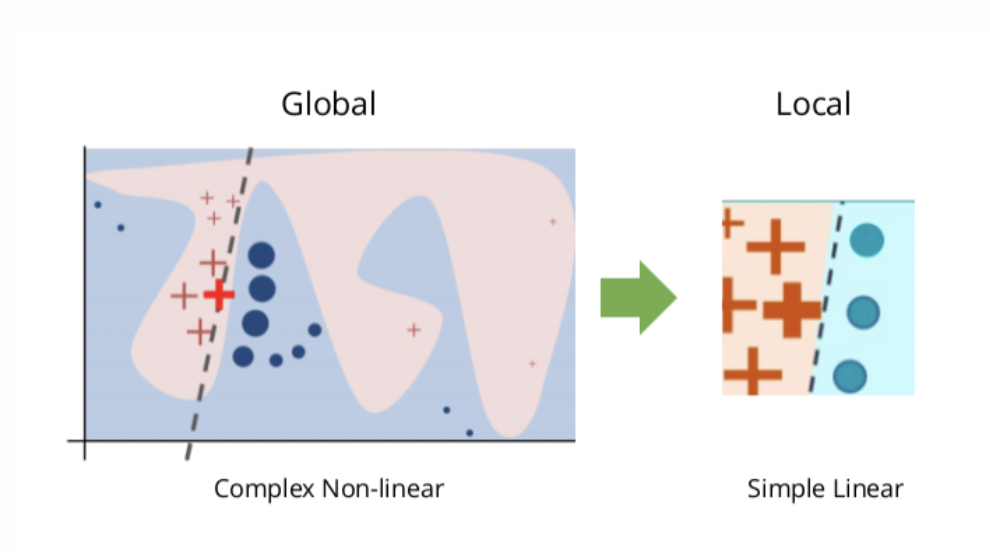LIME, or вАЬLocal Interpretable Model-Agnostic Explanations,вАЭ is an explainability method that attempts to provide local ML explainability. At a high level, LIME attempts to understand how perturbations in a modelвАЩs inputs affect the end-prediction of the model. Since it makes no assumptions about how the model reaches the prediction, it can be used with any model architecture, hence the вАЬmodel-agnosticвАЭ part of LIME. The LIME explainability approach takes a single input value of predictions and perterbs the inputs around those values. It then builds a linear model off of the feature perturbations where the coefficients are the feature importances at this local prediction.

### Logarithmic Loss

вАЛLog-Loss Tracks incorrect labeling of the data class by the model and penalises the model if deviations in probability occur into classifying the labels. Low log loss values equate to high accuracy values.

### MAE

Mean Absolute Error is a regressive loss measure looking at the absolute value difference between a modelвАЩs predictions and ground truth, averaged out across the dataset. Unlike MSE, MAE is weighted on a linear scale and therefore doesnвАЩt put as much weight on outliers. This provides a more even measure of performance, but means large errors and smaller errors are weighted the same. Something to consider depending on your specific model use case.
вАЛ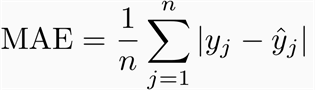вАЛ

### MAP@K

вАЛMAP (Mean Average Precision) @K is a metric used to evaluate the performance of a ranking model. MAP weighs errors to account for value differences between the top and bottom of the list but is limited to binary relevancy (relevant/non-relevant) and can not account for order-specific details.
The higher the MAP@K score, the better the ranking algorithm or recommendation system performs.Precision is the fraction of relevant items among the total number of items returned by the system. Average precision is the average of the precision values at each position where a relevant item is retrieved.MAP @ K=5, calculation for a ranking model across 3 searches

### MAPE

вАЛMean Absolute Percentage Error is one of the most common metrics of model prediction accuracy and the percentage equivalent of MAE. MAPE measures the average magnitude of error produced by a model, or how far off predictions are on average. See MAE for considerations when using this metric.### MASE

Mean absolute scaled error (MASE) is an accuracy metric for forecasting. It is the mean absolute error of the forecast values, normalized by the naive forecast. The naiћИve forecast refers to a simple forecasting method that uses the demand value of a previous time point as the forecast for the next time point. A lower MASE is considered to have higher accuracy.
вАЛвАЛ

### MRR (Mean Reciprocal Rank)

MRR (Mean Reciprocal Rank) is a metric used to evaluate the performance of a ranking model. MRR is the summation of relevant predictions within a list divided by the total number of recommendations.
MRR calculates the mean of the first relevant recommendation, evaluating how well your algorithm predicts your first relevant item.### MSE

Mean Square Error a regressive loss measure. The MSE is measured as the difference between the modelвАЩs predictions and ground truth, squared and averaged out across the dataset. It is used to check how close the predicted values are to the actual values. As in RMSE, a lower value indicates a better fit, and it heavily penalizes large errors or outliers.
вАЛ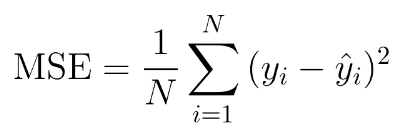вАЛ

### Model Performance

The performance of a machine learning model indicates its usability and ability to provide accurate results. Performance is usually measured in terms of metrics that apply to the specific type of machine model concerned. Here are some common metrics used according to the type of machine model:
• Regression based machine learning models - MSPE, MSAE, R Squared and Adjusted R Squared
• Classification - Precisions-Recall, ROC-AUC, Accuracy, log-loss
• Unsupervised models - Rand index, Mutual information

### Model Store

A machine learning infrastructure tool that serves as central model registry and track experiments. Think of them as the library or catalog of your models. Model stores are used to:
• Serve as a central repository of all models and model versions
• Allow for reproducibility of every model version
• Track lineage of models history

### Monitor Threshold

Monitor threshold refers to the value set for a model monitor, beyond which the modelвАЩs monitoring status will be triggered accordingly. The threshold value can be set on any specific performance metric such as accuracy, MSE, MAPE, etc.

### NDCG

вАЛNDCG measures a model's ability to rank query results in the order of the highest relevance. Actual relevance scores are usually determined by user interaction. For example, if users tend to click on results ranked high on the list, then the NDCG value will be high. Conversely, if users tend to click on query results that are ranked low on the list, it would mean that the ranking model is doing poorly, and the NDCG value will be low. NDCG values range between 0 and 1 with 1 being the highest. Arize computes NDCG using the standard log2 discount function.

### Natural Language Processing (NLP)

Natural language processing (NLP). The inputs to these models are typically sentences, such as: вАЬThis definition is so informative.вАЭ These inputs are broken up into tokens: вАЬThisвАЭ вАЬdefinitionвАЭ вАЬisвАЭ вАЬsoвАЭ вАЬinformative.вАЭ Most commonly, a classification model runs on top of NLP.

### Percentiles

Percentiles help you understand your data quality to account for outlier events and gain a more representative understanding of your data. The Arize platform supports P50, P95, and P99 for data quality monitors:
• P50 - Median data performance
• P95 & P99 - Outlier data performance

### Performance Impact Score

Performance Impact Score indicates how a particular slice is performing relative to the average performance, weighted by volume. This is a proprietary metric created by Arize.
Slice Performance Impact = (Slice Performance - Overall Model Performance) * % of Volume The idea here is that you want to understand which slices are performing best or worst, and weight this difference by volume. Performance is weighted by volume because the more volume a particular slice is seen, the more of an impact it can have on the overall performance.

### Performance Slice

A performance slice is a subset of model values of interest in performance analysis and troubleshooting. Slices can be formed from any model dimension, including specific periods of time, set of features, etc. Performance slice analysis is useful when the goal is to understand or troubleshoot a cohort of interest, such as with bias detection, where the generalized dataset might mask statistical nuances.

### Population Stability Index (PSI)

вАЛPopulation Stability Index looks at the magnitude which a variable has changed or shifted in distribution between two samples over the course of a given time. PSI is calculated as:
PSI = (%Production - %Baseline) x ln(%Production / %Baseline)
The larger the PSI, the less similar your distributions are, which allows you to set up thresholding alerts on the drift in your distributions. PSI is a great metric for both numeric and categorical features where distributions are fairly stable.

### PR AUC Curve

The Precision-Recall AUC curve is the correlation between the precision and recall at particular cut-off values, with the cut off values being set according to the particular model.

### Precision

вАЛPrecision is the fraction of values that actually belong to a positive class out of all the values which were predicted to belong to that class
Precision = True Positives / (Predicted True Positives + Predicted False Positives)
Example: There are 100 credit card transactions; 80 transactions are legitimate (positive class) and 20 transactions are fraudulent. If your model predicts that 85 transactions are legitimate, its precision is:
94.12% = 80 true positives / (80 true positives + 5 false positives)

### Prediction Drift Impact

The product of feature importance and drift (PSI -- population stability index).
Prediction Drift Impact = Feature Importance * Drift

### Quantile

Quantiles are the points dividing the range of a probability distribution into intervals with equal probabilities.

### Ranking Approaches

Ranking approaches differ in how many items you consider at a time in training.

#### Pointwise - Single Item

The total loss is computed as the sum of loss terms defined on each item as the distance between the predicted score and the ground truth. This transforms our task into a classification/regression problem, where we train a model to predict y.

#### Pairwise - Pair of Items

The total loss is computed as the sum of loss terms defined on each pair of items. The objective is to predict which two items are more relevant, then compare the prediction to ground truth. This transforms the task into a binary classification problem.

#### Listwise - List of Items

Loss is directly computed on an entire list of items with respective ranks вАУ where ranking metrics are directly related to loss.

### Recall

вАЛRecall is the fraction of values predicted to be of a positive class out of all the values that truly belong to the positive class (including false negatives)
recall = predicted true positives / (true positives + false negatives)

### Recall Parity

Recall Parity is defined as the Recall of a sensitive group as compared to the recall of a base group.### ROC - AUC

The Receiver Operating Characteristics (ROC) is a probability curve plotted between true positive rate (TPR) and false positive rate (FPR). Area Under the Curve (AUC) is an aggregate measure of performance across all possible classification thresholds. Together, ROC - AUC represents the degree of separability, or how much a model is capable of distinguishing between classes.
The higher the AUC (i.e. closer to 1), the better the model is at predicting 0 class as 0, and 1 class as 1.
FPR = X = fp / ( tn + fp) => error: when all actuals are negative
TPR = Y = tp / (tp + fn) => error: when all actuals are positive
вАЛ
вАЛвАЛ
вАЛ
To calculate AUC/PR AUC: Threshold: We first need to generate a confusion matrix based on the threshold. There are 20 thresholds by default , so there will be 20 confusion matrices as well.
From each confusion matrix: we calculate the False Positives Rate (x-axis) and True Positive Rate (y-axis) for AUC. As a result, we will have a set of 20 points (x and y coordinates) for AUC or PR-AUC.
вАЛAUC: The next step is to calculate the area under the curve (AUC) for the 20 points. We first sort the points in order of x-axis increasing, and then a secondary sort on y-axis to make it consistent between runs. According to trapezoidal rule, we find the difference between x values of two consecutive points as delta x, and multiply by the average of y values of two consecutive points to get the area between two consecutive points. Lastly, we do the cumulative sum for all 20 points to get the overall AUC.

### Regression

Regression analysis is a fundamental concept in data science and machine learning. It helps quantify the relationship between the inputs into a model and its outputs. Essentially, it is an estimation of how a variable affects a set of independent variables.

### RMSE

Root Mean Square Error (also known as root mean square deviation, RMSD) is a measure of the average magnitude of error in quantitative data predictions. It can be thought of as the normalized distance between the vector of predicted values and the vector of observed (or actual) values.
вАЛвАЛ
Because errors are squared before averaged, this measure gives higher weight to large errors, and therefore useful in cases where you want to penalize models accordingly.

### Score Models

Score models generate a numeric value as its prediction or output. For example, the likelihood that an input belongs to a category.

### Sensitivity

Sensitivity is a measure of the number of positive cases that turned out to be true for a given model. It is also called the true positive rate.
sensitivity = predicted true positives / (true positives + false negatives)

A method of testing a candidate model for production where production data runs through the model without the model actually returning predictions to the service or customers. Essentially, simulating how the model would perform in the production environment.

### SHAP

вАЛSHAP stands for вАЬShapley Additive Explanations,вАЭ a concept derived from game theory and used to explain the output of machine learning models (see definition of вАШExplainabilityвАЩ). SHAP values help interpret how much a given feature or input contributes, positively or negatively, to the target outcome or prediction. See вАШFeature ImportanceвАЩ

### sMAPE

Symmetric Mean Absolute Percentage Error (sMAPE) is an accuracy metric based on percentage. By dividing by both actual and predicted values and normalizing the relative errors, sMAPE overcomes the asymmetric shortcomings found in MAPE. sMAPE ranges with a lower bound of 0% and an upper bound of 200% (in the Arize platform this is reflected from 0-->2), which enables models that have forecasts higher than actuals to attain more accurate negative percentage error approximations.### Specificity

Specificity is the fraction of values predicted to be of a negative class out of all the values that truly belong to the negative class (including false positives). This measure is similar to recall, but describes the offset in correcting predicting negative values. It is also called the true negative rate.
specificity = predicted true negatives / (true negatives + false positives)
Example: There are 100 credit card transactions; 90 transactions are legitimate and 10 transactions are fraudulent (negative class). If your model predicts that 20 transactions are fraudulent, its recall is:
50% = 10 true negatives / (10 true negatives + 10 false positives)

### Tabular Data

Data in a table format, with columns and rows. Inputs of the model in a table format (i.e. an Excel spreadsheet), where columns might be feature inputs (i.e city, state, charge amount). NLP and images do not fit in an excel sheet, since inputs are sentences or images.

### Tag

A tag is used to store extra information or metadata alongside a prediction. They are different than features in that they are not actual inputs in the model.

### True Negative

When a model correctly predicts a negative class, when the value belongs to the negative class.
Example: A model flags a credit card transaction as вАШnot fraudвАЩ when it is actually a legitimate transaction.

### True Positive

When a model correctly predicts a positive class, when the value belongs to the positive class.
Example: A model flags a credit card transaction as вАШfraudвАЩ when it is actually a fraudulent transaction.

### WAPE

Weighted Average Percentage Error, also referred to as the MAD/Mean ratio. The WAPE metric is the sum of the absolute error normalized by the sum of actual values. WAPE equally penalizes for under-forecasting or over-forecasting, and does not favor either scenario.
WAPE = sum(absError)/sum(Actuals)
When the total number of sales can be low or the product analyzed has intermittent sales, WAPE is recommended over MAPE. MAPE is commonly used to measure forecasting errors, but it can be deceiving when sales reach numbers close to zero, or in intermittent sales (referenced here). WAPE is a measure that counters this by weighting the error over total sales. WAPE is more robust to outliers than Root Mean Square Error (RMSE) because it uses the absolute error instead of the squared error.

### Worst Performing Slice

When using the worst performing slice tool inside of Arize, we can understand which slices have the most affect on the our overall performance. Here we can see the percentage difference between the slice and our overall model performance divided by the overall performance. Note this calculation is accounting for volume of particular slices inherently.
Worst Performing Slice = (Performance Excluding Slice - Overall Performance) / Overall Performance) * 100